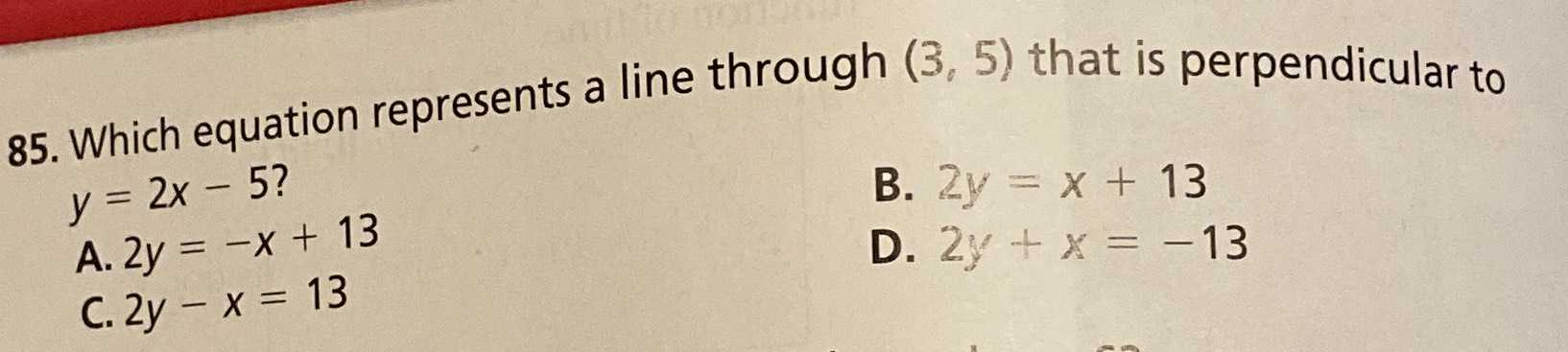### ¿Todavía tienes preguntas de matemáticas?

Pregunte a nuestros tutores expertos
Algebra
Pregunta85. Which equation represents a line through $$( 3,5 )$$ that is perpendicular to

$$y = 2 x - 5 ?$$ B. $$2 y = x + 13$$

A. $$2 y = - x + 13$$ D. $$2 y + x = - 13$$

C. $$2 y - x = 13$$